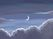cancel
Showing results for
Did you mean:

## Calculation as per month

Hello Team,

i am having 3 columns
1. Dimension date column
2. Count as per month.
3. Total cost per month.

my requirment is i want to calculate
eg
Jan count divide by january cost.
Feb + Jan Count divide by February cost.

What measure should i use can anybody please suggest.

Best Regards,
Sagar Shah

Status: Delivered

``````measure=

var _a = calculate(count('table'[count]),filter(all(table),'table'[date]<=eomonth(max('table'[date]))))

var _b = sum('table'[cost])

return divide(_a,_b)``````

Best regards.
Community Support Team_ CaitlynCommunity Support
Status changed to: Delivered

``````measure=

var _a = calculate(count('table'[count]),filter(all(table),'table'[date]<=eomonth(max('table'[date]))))

var _b = sum('table'[cost])

return divide(_a,_b)``````

Best regards.
Community Support Team_ CaitlynFrequent Visitor

Hey Caitlyn,

I tried to utilize the formula but not working,

Can you show me one use case it would be helpfull.

Thanks,
Sagar Shah

Completed Ideas